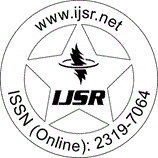International Journal of Science and Research (IJSR)
Call for Papers | Fully Refereed | Open Access | Double Blind Peer Reviewed

Research Paper | Mathematics | India | Volume 6 Issue 3, March 2017

# A Deterministic Inventory Model with Selling Price Dependent Demand Rate, Quadratic Holding Cost and Quadratic Time Varying Deteriorating Rate

Varsha Sharma  | Anil kumar Sharma 

Abstract: In this paper we consider an inventory model for deteriorating products having demand which is function of selling price, here the deterioration rate is time varying with quadratic function of time. Holding cost is considered as a quadratic function of time. This model is developed to find the total cost of the inventory system. Suitable numerical example and sensitivity analysis also discussed. At the end of the paper, the optimum total cost and total order quantity has been calculated by the change of the value of the each parameter and graphs shows the behavior of the relation between parameter and total inventory cost.

Keywords: Inventory, demand, Selling Price, Deteriorating items, Holding Cost Optimum cost

Edition: Volume 6 Issue 3, March 2017,

Pages: 193 - 198